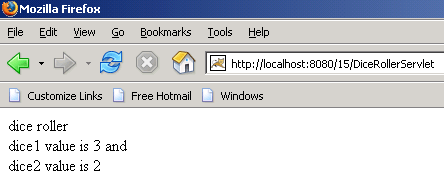Tutorials

# Dice Roller

We are going to make one program on the dice roller in which the number in the dice will be selected randomly.

We are going to make one program on the dice roller in which the number in the dice will be selected randomly.

# Dice Roller

We are going to make one program on the dice roller in which the number in the dice will be selected randomly.

To make a program over this firstly we need to make a class DiceRoller in which we will have a doGet() method in which we will have our application logic. To make the dice working randomly use the random() method of the class java.lang.Math. To print the number on the browser call the method getWriter() of the response object which will return the PrintWriter object. Now by the object of the PrintWriter class print the values of the dice on the browser.

The code of the program is given below:

 ``` import java.io.*; import javax.servlet.*; import javax.servlet.http.*; public class DiceRollerServlet extends HttpServlet{ protected void doGet(HttpServletRequest request, HttpServletResponse   response)throws ServletException, IOException{   PrintWriter pw = response.getWriter();   String dice1 = Integer.toString((int)(Math.random()*6)+1);   String dice2 = Integer.toString((int)(Math.random()*6)+1);   pw.println("");   pw.println("dice roller
");   pw.println("dice1 value is " + dice1 + " and
dice2 value is "    +dice2); } }```

XML File for this program:

 ```    Zulfiqar  DiceRollerServlet      Zulfiqar  /DiceRollerServlet   ```

The output of the program is given below: